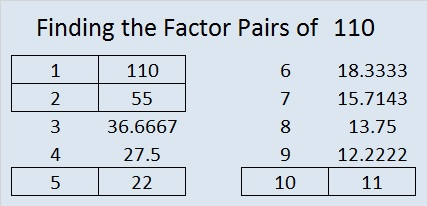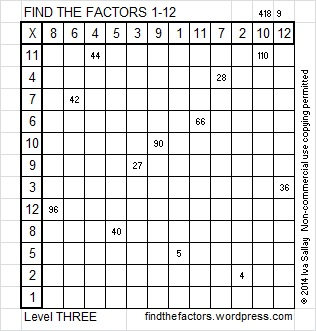# 110 and Level 3

110 is a composite number. 110 = 1 x 110, 2 x 55, 5 x 22, or 10 x 11. Factors of 110: 1, 2, 5, 10, 11, 22, 55, 110. Prime factorization: 110 = 2 x 5 x 11.When 110 is a clue in the FIND THE FACTORS 1 – 12 puzzles, use 10 and 11 as the factors.Excel file of puzzles and the previous week’s solutions: 12 Factors 2014-05-05

A Logical Approach to FIND THE FACTORS: Find the column or row with two clues and find their common factor. Write the corresponding factors in the factor column (1st column) and factor row (top row).  Because this is a level three puzzle, you have now written a factor at the top of the factor column. Continue to work from the top of the factor column to the bottom, finding factors and filling in the factor column and the factor row one cell at a time as you go: